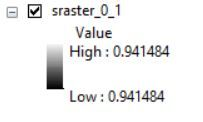# Export values of rasters without attribute table

390
9
11-28-2020 04:35 AM

Hello, everyone !

I have a file with multiple rasters (stretched - without attribute table - but with only one value e.g. min value = max value) and I am trying to export each raster's value in a table/xls file where the values from all the rasters will have been registered (in a column for example) using model builder or arcpy.

Any suggestion is welcomed, thank you !

2 Solutions

Accepted Solutions
byMVP Regular Contributor

How many rasters have you got? You're saying each raster is made of cells entirely of one value?

Can you post a picture of this?

``````raster_object = arcpy.Raster(r'C:\MyStuff\Geo\Raster1.tif)
min_value = raster_object.minimum
max_value = raster_object.maximum``````

byMVP Frequent Contributor
``````import arcpy
import numpy as np

arcpy.env.workspace = "c:/data/DEMS"  # --- Set the current workspace
rasters = arcpy.ListRasters("*")
out = []
for raster in rasters:
inRas = arcpy.Raster(raster)
arr = arcpy.RasterToNumPyArray(inRas)
ras_min = np.min(arr)
out.append([raster, ras_min])
print(out)

# ---- for now, just print it or see
# NumPyArrayToTable``````

Now, if you have nodata values to account for, then the code will have to be edited, or if the rasters are all over the place, then good luck.

This should give you an idea... but I never tested it, just cobbled together from the help files

NumPyArrayToTable—ArcGIS Pro | Documentation

... sort of retired...
9 Replies
byMVP Regular Contributor

How many rasters have you got? You're saying each raster is made of cells entirely of one value?

Can you post a picture of this?

``````raster_object = arcpy.Raster(r'C:\MyStuff\Geo\Raster1.tif)
min_value = raster_object.minimum
max_value = raster_object.maximum``````

I have 1176 rasters. Yes, each raster is made of cells entirely of one value.byMVP Regular Contributor

try the above script on one of the rasters.  If that works, it can easily be put into a for loop to iterate over the 1000.  Unsure if it's the fastest way if you're looking to do this regularly? Maybe @DanPatterson has a numpy method.

Thank you, I' ll try it.. Should I add an 'export' expression after your script so as to save the value in a new table/array ?

byMVP Regular Contributor

for the moment just use

print(min_value)

You can read min values from the each raster and add to a list, then write into an excel file. You might need to download xlswriter library or write into .dbf file and convert to excel file. There are multiple ways writing from list to excel file

`import arcpyimport osimport xlsxwriterraster_list = os.listdir(path/to/rasterfolder)value_list = []for each_raster in raster_list:    result = arcpy.GetRasterProperties_management(each_raster, "MINIMUM")    min_value = result.getOutput(0)    value_list.add(min_value)with xlsxwriter.Workbook('RasterValues.xlsx') as workbook:    worksheet = workbook.add_worksheet()    for row_num, data in enumerate(value_list):        worksheet.write_row(row_num, 0, data)  `

Thank you, I tried to install xlsxwrite with several ways but "invalid syntax" message is always appeared..

byMVP Frequent Contributor
``````import arcpy
import numpy as np

arcpy.env.workspace = "c:/data/DEMS"  # --- Set the current workspace
rasters = arcpy.ListRasters("*")
out = []
for raster in rasters:
inRas = arcpy.Raster(raster)
arr = arcpy.RasterToNumPyArray(inRas)
ras_min = np.min(arr)
out.append([raster, ras_min])
print(out)

# ---- for now, just print it or see
# NumPyArrayToTable``````

Now, if you have nodata values to account for, then the code will have to be edited, or if the rasters are all over the place, then good luck.

This should give you an idea... but I never tested it, just cobbled together from the help files

NumPyArrayToTable—ArcGIS Pro | Documentation

... sort of retired...

Thank you, I replaced 'min' with 'max' and it returned the right values, because when I run it for 'min' it returned numbers tending to zero.